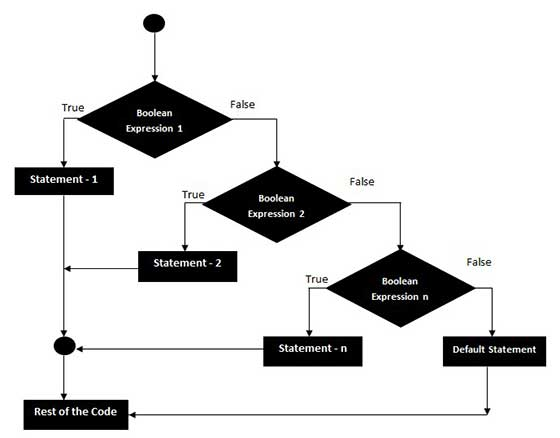# VBScript If..ElseIf..Else Statements

An If statement followed by one or more ElseIf Statements that consists of boolean expressions and then followed by a default else statement, which executes when all the condition becomes false.

## Syntax

The syntax of an If-ElseIf-Else statement in VBScript is −

```If(boolean_expression) Then
Statement 1
.....
.....
Statement n
ElseIf (boolean_expression) Then
Statement 1
.....
....
Statement n
ElseIf (boolean_expression) Then
Statement 1
.....
....
Statement n
Else
Statement 1
.....
....
Statement n
End If
```

## Flow Diagram## Example

```<!DOCTYPE html>
<html>
<body>
<script language = "vbscript" type = "text/vbscript">
Dim a
a = -5

If a > 0 Then
Document.write "a is a POSITIVE Number"
ElseIf a < 0 Then
Document.write "a is a NEGATIVE Number"
Else
Document.write "a is EQUAL than ZERO"
End If
</script>
</body>
</html>
```

When the above code is executed, it produces the following result −

```a is a NEGATIVE Number
```
vbscript_decisions.htm